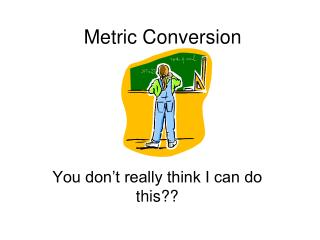# Metric Conversion - PowerPoint PPT PresentationDownload PresentationMetric Conversion

Metric ConversionDownload Presentation## Metric Conversion

- - - - - - - - - - - - - - - - - - - - - - - - - - - E N D - - - - - - - - - - - - - - - - - - - - - - - - - - -
##### Presentation Transcript

1. Metric Conversion You don’t really think I can do this??

2. Introduction to the Metric System:There are four standard units of metric measurement.

3. 1. These units will be used to measure length, volume, mass, and temperature. a. The unit for length is the ... meter b. The unit for volume is the ... liter. c. The unit for mass is the … gram. d. The unit for temperature is ... celsius.

4. There are prefixes that can be attached to meter, liter, and gram that will change the value of the unit making it either larger or smaller. 1. The prefix kilo- increases the standard unit by 1,000 times. 1000 meters = 1 kilometer 2. The prefix deci- decreases the standard unit by 10 times. 1 liter = 10 deciliters 3. The prefix centi- decreases the standard unit by 100 times. 1 gram = 100 centigrams

5. 4. The prefix milli- decreases the standard unit by 1,000 times. 1 meter = 1,000 millimeters 5. The prefix micro- decreases the standard unit by1,000,000 times. 1 liter = 1,000,000 microliters

6. The Metric Staircase A staircase is an excellent model of how the prefixes of metric units relate to each other.

7. The Metric Staircase Kilo 1,000 Hecto 100 Deka 10 Ones/Base 1 Deci 1/10 or 0.1 Centi 1/100 or 0.01 Milli These are the prefixes that apply to all metric units. 1/1000 or 0.001

8. Kilo Kids Hecto Have Deka Dropped Ones Over Deci Dead Centi Changing Milli Metrics Here is a sentence to help you remember the prefixes.

9. Length Kilometer km Hectometer hm Dekameter dkm Meter m Decimeter dm Centimeter cm Larger Millimeter mm The standard unit of length is the meter.

10. Mass Kilogram kg Hectogram hg Dekagram dkg Gram g Decigram dg Centigram cg Larger Milligram mg The standard unit of mass is the gram.

11. Volume Kiloliter kl Hectoliter hl Dekaliter dkl Liter l Deciliter dl Centiliter cl Larger Milliliter ml The standard unit of volume is the liter.

12. Kilo Hecto Deka Ones/Base Deci Centi Milli The Metric Staircase The staircase also tells us which way to move the decimal. As the unit gets smaller, you shift the decimal to the right. As the unit gets larger, the decimal shifts to the left.

13. Kilo Hecto Deka Ones/Base Deci Centi Milli The Metric Staircase The metric staircase is easy to use because each unit differs from the next by a factor of 10. Units get larger as you climb. Units get smaller as you descend.

14. Kilo Hecto Deka Ones/Base Deci Centi Milli The Metric Staircase When converting between units, move the decimal one place for every step taken. Move it in the direction shown on the staircase. If there is not a number in the moved place, place a zero in the spot.

15. km hm dkm m dm cm mm Let’s use a line to represent a distance of a kilometer. 1 km=10 hm=100 dkm=? m = ? dm = ?cm= ? mm

16. Sample Problem: 7.359 m = ???? cm km hm dkm m dm cm mm To get from m to cm, we must take 2 steps to the right. Therefore, we must shift the decimal two places to the right. . Answer: 735.9 cm 7.359 m = 7 . 3 5 9 cm

17. Sample Problem: 1200 m = ???? km km hm dkm m dm cm mm . . 1200 m = 1 2 0 0 km Answer: 1.2 cm

18. 45.6 cm = ?? m

19. 45.6 cm = .456 m

20. 4568 g = ?? kg

21. 4568 g = 4.568 kg

22. 9.08 l = ?? ml

23. 9.08 l = 9,080 ml

24. 320 mg = ?? g

25. 320 mg = 0.320 g

26. Homework 1) 10 L = ?? ml 2) 123 mm = ?? cm 3) 3000 g = ?? kg

27. 5) 1.2 kg = ?? dg 4) 1.2 kg = ?? dkg 6) 5 g = ?? mg 7) 50 g = ?? kg